Next: Transformation of Laplace Tidal Up: Terrestrial Ocean Tides Previous: Non-Global Ocean Tides

# Useful Lemma

Let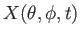,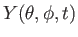,, andbe well-behaved functions of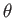and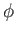. Suppose that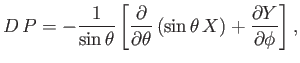(12.186)

where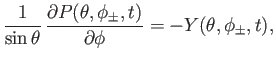(12.187)

Suppose, further, that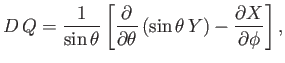(12.188)

where(12.189)

We wish to demonstrate that(12.190)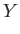(12.191)

Let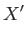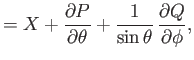(12.192)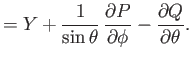(12.193)

Note, from Equations (12.192) and (12.194), that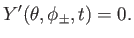(12.194)

It follows from Equations (12.191), (12.193), (12.197), and (12.198) that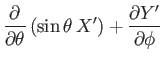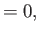(12.195)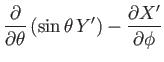(12.196)

Equations (12.199) and (12.201) imply that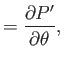(12.197)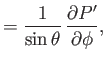(12.198)

and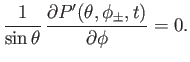(12.199)

Furthermore, Equation (12.200) yields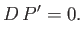(12.200)

Multiplying by, integrating over the ocean, and making use of the boundary condition (12.204), we obtain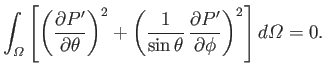(12.201)

Here,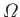is the surface of the Earth that is covered by ocean, and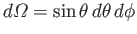. It follows thatis a constant. Thus, Equations (12.202) and (12.203) imply that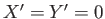, and, hence, that Equations (12.195) and (12.196) are valid.Next: Transformation of Laplace Tidal Up: Terrestrial Ocean Tides Previous: Non-Global Ocean Tides
Richard Fitzpatrick 2016-03-31